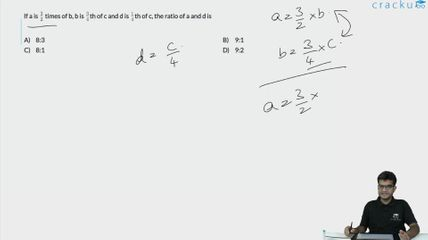Question 5

# If a is $$\frac{3}{2}$$ times of b, b is $$\frac{3}{4}$$th of c and d is $$\frac{1}{4}$$th of c, the ratio of a and d is

Solution

$$a=\frac{3}{2}b$$
b=$$\frac{3}{4}c$$ and
d=$$\frac{c}{4}$$
c=4d
Now substituting we get
$$a=\frac{3}{2}\ \times\ \frac{3}{4}\times\ 4d$$
so we get a=$$a=\frac{9}{2}d$$
a:d=9:2

### View Video Solution